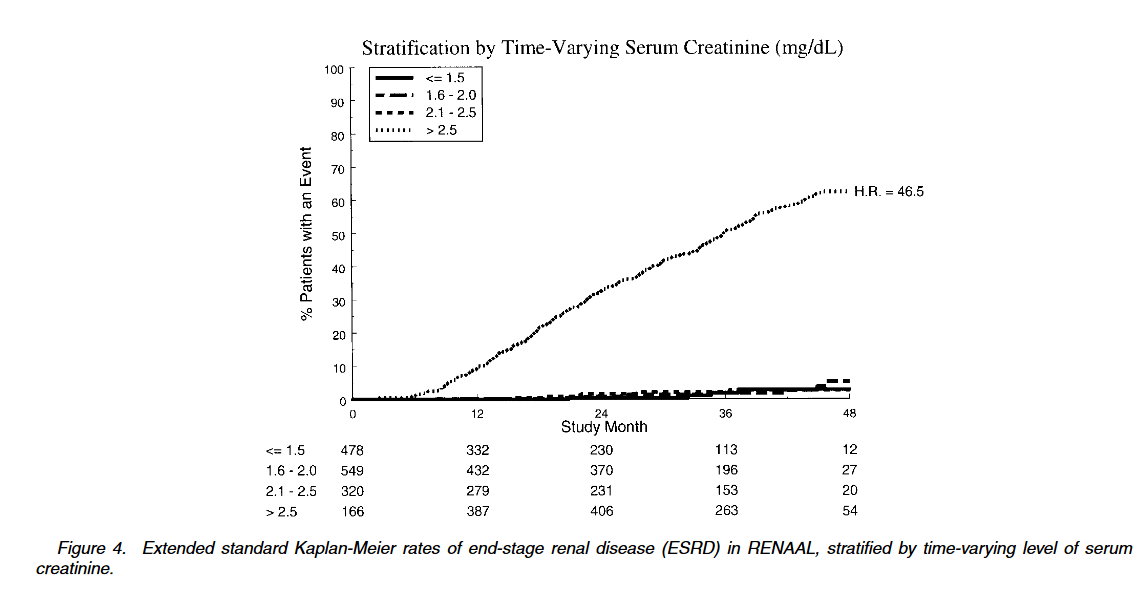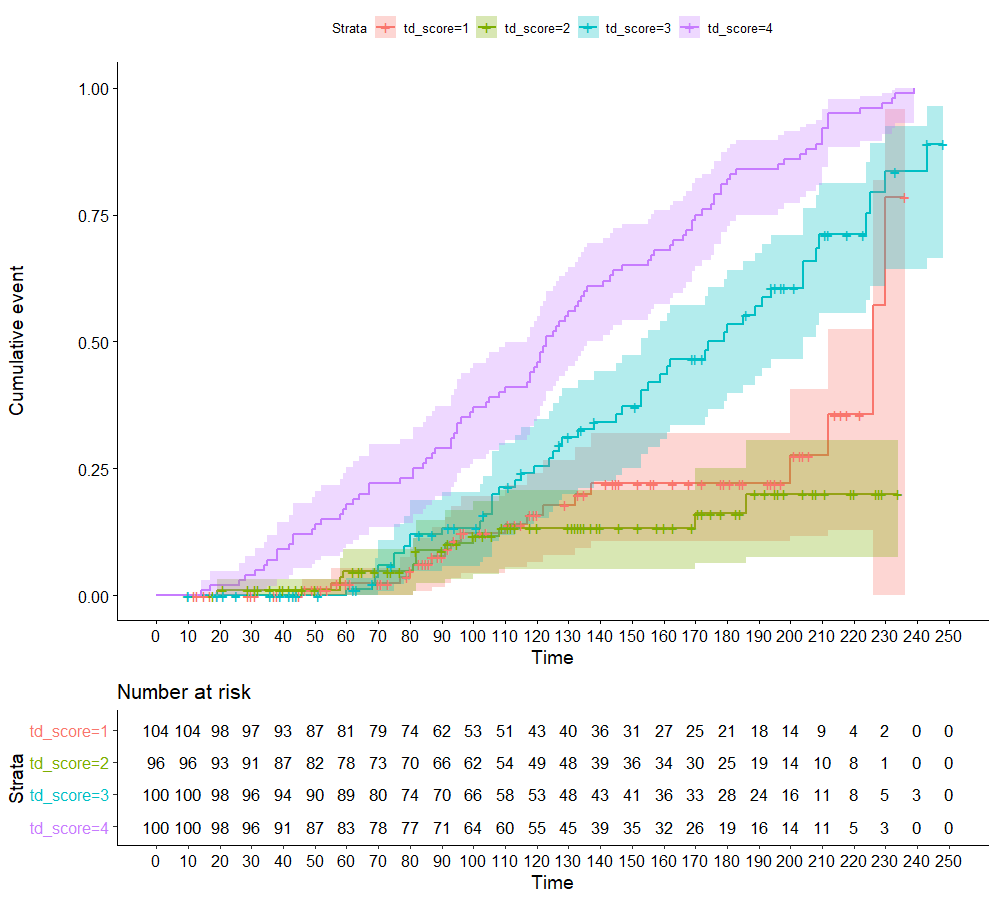# #StackBounty: #r #survival #cox-model #kaplan-meier Extended Kaplan-Meier for time-dependent covariates

### Bounty: 50

I have read Snapinn et al. paper on "Illustrating the Impact of a Time-Varying Covariate With an Extended Kaplan-Meier Estimator" (https://doi.org/10.1198/000313005X70371). They describe an extended Kaplan-Meier survival analysis for working with time-dependent covariates. Please reach out if you need the full text article.I have been trying to replicate their results but I am reaching out to you to validate my results. Since they haven’t made their data available, I made up some data and I already ran the following in R:

``````library(survival)
library(survminer)
library(tidyverse)

set.seed(99)

#arbitrary data
df1 <- data.frame(ID = rep(seq(1, 400, by = 1), 2),
score = factor(sample(1:4, 200, replace = TRUE)),
timetoFU = sample(1:200, 200, replace = TRUE),
status = sample(c(0, 1), 200, replace = TRUE, prob = c(0.9, 0.2))
)

df1 <- df1 %>% group_by(ID) %>% arrange(ID, timetoFU) %>% mutate(obs_n = row_number(), time_max = last(timetoFU)+sample(1:50, 1, replace = TRUE)) %>% ungroup()
df1$$timetoFU[which(df1$$obs_n == 1)] <- 0
#events more likely with higher scores
temp <- df1 %>% group_by(ID) %>% summarise(risk=sum(as.numeric(score))/10, status, time_max, obs_n) %>% ungroup() %>% mutate(status = ifelse(risk >0.4, sample(c(0,1), 100, replace = TRUE), status)) %>% mutate(status = ifelse(risk > 0.6, 1, status)) %>% filter(obs_n == 1)

#build time dependent variable data frame
td_df <- tmerge(temp, temp, id = ID, outcome = event(time_max, status))
td_df <- tmerge(td_df, df1, id = ID, td_score = tdc(timetoFU, score))

#survival analysis

s1 <- survfit(Surv(tstart, tstop, outcome) ~  td_score, data = td_df, id = ID)
ggsurvplot(s1, fun = "event", risk.table = TRUE, conf.int = TRUE, break.x.by = 10)

cox_fit_A <- coxph(formula = Surv(tstart, tstop, outcome) ~ td_score, data = td_df, id = ID)
summary(cox_fit_A)
``````Is this correct?

Get this bounty!!!

This site uses Akismet to reduce spam. Learn how your comment data is processed.